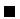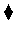Next: The Execution Mechanism Up: A Basic Language Previous: Searching

# Logical Variables

Variables in CLP languages are termed logical variables. The adjective logical stems from a unique character not present in other languages: these variables do not necessarily hold values--and yet they are completely legal, and run-time access exception errors are not generated by accessing them2.2--, and they can be assigned (or, better, bound) to other uninitialized variables. The value of an uninitialized variable is not NULL or other esoteric, special value: that variable, simply, has no value at all yet.

Logical variable assignment is monotonic, which means that a logical variable cannot mutate its value within a search path.

Example 2.9   The variable X can take the value a:

```?- X = a.
X = a
```

But it cannot take the value a and then change it to b

```?- X = a, X = b.
no
```Problem 2.2   Then, how is it possible that the following queries work perfectly?

```?- X = a.
X = a
?- X = b.
X = b
```

Hint: the toplevel interpreter backtracks between goals, in order to recover the initial state.The constraint =/2 we have introduced before not only assigns values to variables (or, better, binds variables to values), but it can also bind free variables, constraining them to have the same value.

Example 2.10   Variables can be bound one to each other, constraining them to take the same value, and this constraint is taken into account during the rest of the execution:

```?- X = Y, X = a.
X = a, Y = a.

?- X = Y, pet(X).
X = spot, Y = spot ;
X = barry, Y = barry
```Problem 2.3   Explain the following behavior: why the query has no solutions?

?- X = Y, pet(X), sound(Y, roar).
noProblem 2.4   Given the following program, which is intended to model kinship in a family:

```father_of(juan, pedro).
father_of(juan, maria).
father_of(pedro, miguel).
mother_of(maria, david).
grandfather_of(L,M):-
father_of(L,N),
father_of(N,M).
grandfather_of(X,Y):-
father_of(X,Z),
mother_of(Z,Y).
```

```?- father_of(juan, pedro).
?- father_of(juan, david).
?- father_of(juan, X).
?- grandfather_of(X, miguel).
?- grandfather_of(X, Y).
?- X = Y, grandfather\_of(X, Y).
?- grandfather_of(X, Y), X = Y.
```Problem 2.5   Augment the code in Problem 2.4 to contain rules for the relationship
grandmother_of(X, Y), following the spirit of the program.Next: The Execution Mechanism Up: A Basic Language Previous: Searching
MCL
1998-12-03